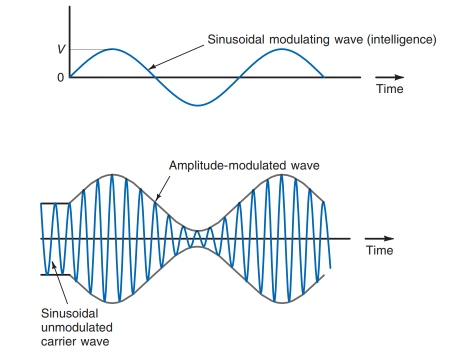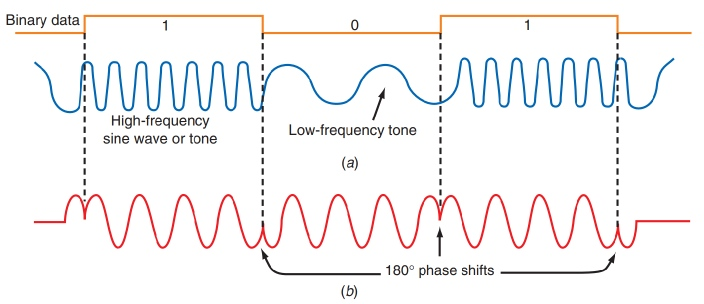# Modulation | Definition, Types and Need for Modulation

The process by which some characteristics of high frequency wave is altered in accordance with the intensity of of the signal is known as modulation.

## Modulation

The process by which some characteristics of high frequency wave such as amplitude, frequency or phase is altered in accordance with the intensity of the signal is known as modulation.

The carrier voltage may be expressed as

ec = Ec cos (ωct + θ)

Where, Ec = peak value of amplitude

ωc = angular frequency

θ = phase angle

## Types of Modulation

### (i) Amplitude Modulation

When the carrier amplitude Ec is varied in accordance with the modulating waves, the process is known as amplitude modulation (AM); carrier frequency ωc and phase angle θ remain constant.Amplitude Modulation

### (ii) Frequency Modulation

When the carrier frequency ωc is varied in accordance with modulating waves, while Ec and θ remain constant, the process is known as frequency modulation (FM).Frequency Modulation

### (iii) Phase Modulation

When the carrier phase angle θ is varied in accordance with the modulating wave, while ωc and Ec remain constant, the process is known as phase modulation (PM).Phase Modulation

## Need for Modulation

Modulation is extremely necessary in communication system due to the following reasons :

### (i) Practical Length of Antenna

Theory shows that in order to transmit a wave effectively, the length of the transmitting antenna should be approximately equal to ¼th time of the wavelength of the wave.

λ=\frac{3×10^{8}}{ν}

For radio frequency range ν = 10 kHz

λ=\frac{30000×10^{4}}{10000}

λ = 30 km

This is too long antenna to be constructed practically and length of antenna is given by

l=\frac{λ}{4}

l=\frac{30000}{4}

l = 7.5 km

Practically, it is impossible to make this height of antenna.

### (ii) Power Radiated by Antenna

The energy of a wave depends upon its frequency. If the frequency of the wave be greater, the greater energy passed by it. Thus, the power radiated increases with increase in frequency. As radio signal frequencies are small, therefore these cannot be transmitted over large distances if power radiated directly into space.

For a good transmission, we need high effective power and it is possible only if we use high frequency transmission. Thus, there is need to translate the audio frequency (AF) baseband signal into radio frequency (RF) frequencies before transmission.

### (iii) Mixing up of signals

In general, many people are talking at the same time. In terms of communication systems, we say that many transmitters are transmitting baseband signals simultaneously and frequencies of all these transmitters lie with audible range below 20 kHz. Obviously, all these signals will get mixed up and there is no simple way to distinguish between the signals from different transmitters. The only solution of the problem is to have communication at high frequencies so that a separate band of frequencies be allotted to each message signal for its transmission.

### (iv) Wireless Communication

One desirable feature of radio transmission is that it should be carried without wires i.e. radiated into space. At audio frequencies radiation is not practicable because the efficiency of radiation is poor. However, efficient radiation of electrical energy is possible at high frequencies (>20 kHz).

The above mentioned four points clearly suggest that there is a need for transmission the original low frequency baseband message/information signal into high frequency wave before transmission such that the transmitted signal continues to possess the information contained in the original message signal. This is achieved by the process of modulation.

## Que: A carrier wave of frequency 12 MHz and peak voltage 30 V is used to modulate in audio signal of frequency 12 kHz and peak voltage of 20 V. Calculate (i) Modulation index, (ii) Side band frequency

Ans: vc = 12 MHz = 12×106 Hz
vm = 12 kHz = 12×103 Hz
Ec = 30 V
Em = 20 V
(i) modulation index ma = Em/Ec
ma = 20/30
ma = 0.66

(ii) U.S.B. = vc + v m = 12000×103 + 12×103
= (12000 + 12)×103
= 12012×103 Hz

L.S.B. = vc - vm
= 12000×103 - 12×103
= (12000 - 12)× 103
= 11988×103 Hz